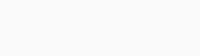Monday, December 4, 2023

# If you want to add a column for conversion value per cost after setting up conversion tracking, how does that get calculated?

-

If you want to add a column for conversion value per cost after setting up conversion tracking, how does that get calculated?

• By dividing the total conversion value by the total cost of all ad interactions
• By dividing the number in the conversions column by the total eligible interactions
• By dividing the total conversion value by the number in the conversions column
• By dividing the total cost by the number in the conversions column